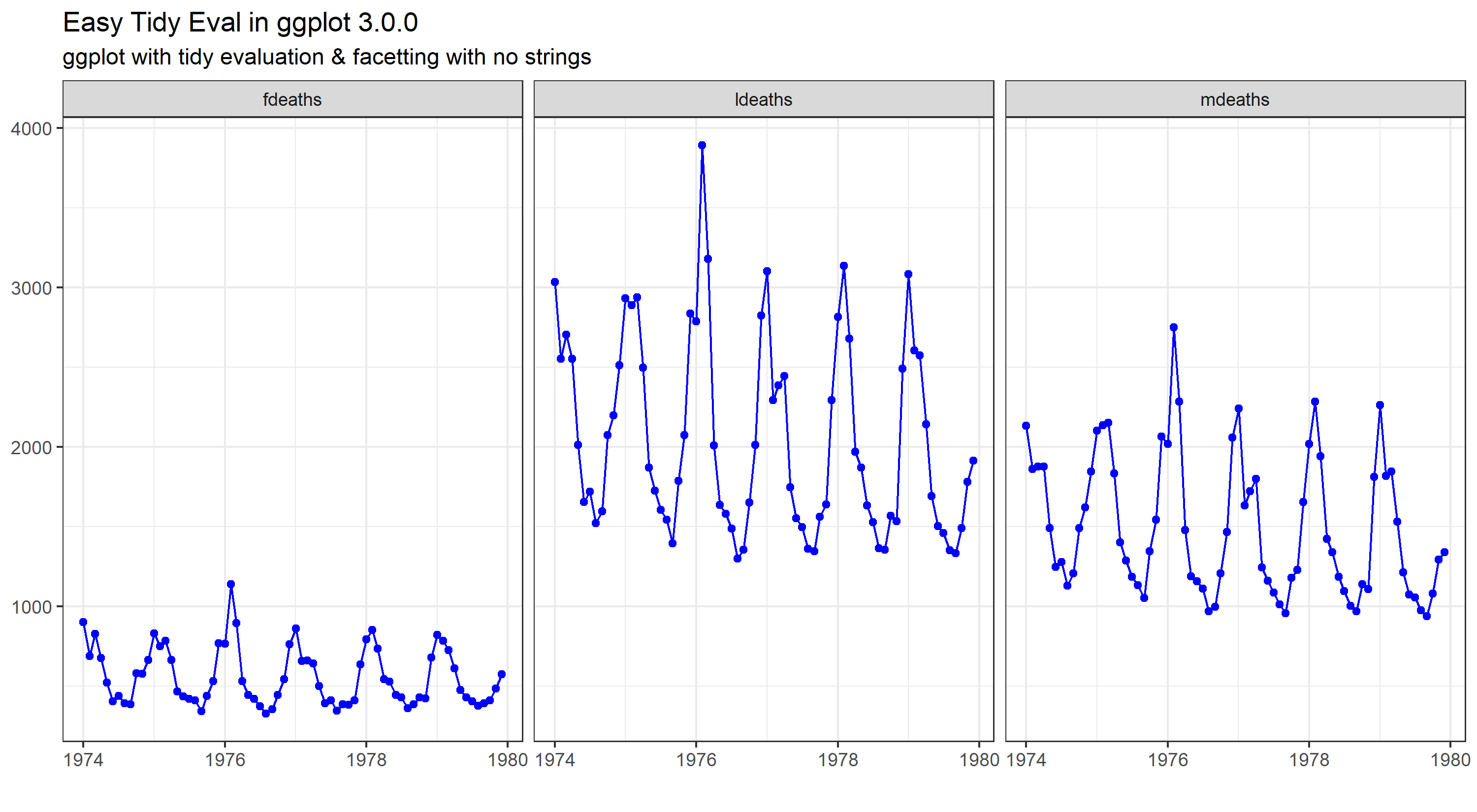# Tidily evaluated ggplot2Test driving tidy evaluation in the new release of ggplot2

A new release of ggplot2

Now that tidy evaluation is baked into ggplot2, as of TODAY, let’s take it for a spin:

ggplot2 3.0.0 %>%
create function %>%
test function %>%
end

library(dplyr)
library(ggplot2)
library(tidyr)
library(tibble)

data <- list(fdeaths, mdeaths, ldeaths) #time series data - needs prep

names(data)[1:3] <- c("fdeaths","mdeaths","ldeaths")

data <- as_tibble(data)

startdate <- as.Date('1974-1-1')

data$date <- seq.Date(startdate,by = 'month',length.out = 72) newdata <- tidyr::gather(data, key = key, value = value,-date) newdata$value <- as.numeric(newdata\$value)

# create generic function

gtest <- function(df,x,y, group) {
x_quo <- enquo(x)
y_quo <- enquo(y)
group_quo <- enquo(group)

p <- ggplot(df,aes(x = !!x_quo, y = !!y_quo)) +   #bangin'
geom_line(colour = "blue", group = 1) +
geom_point(colour = "blue") +
facet_wrap(group_quo, ncol = 3) #look Ma, no need to bang bang here!

p <- p + ggtitle(label = "Easy Tidy Eval in ggplot 3.0.0",
subtitle = "ggplot with tidy evaluation & facetting with no strings")

p <- p + labs(x = NULL, y = NULL, caption = "") +
theme_bw()
print(p)
}


Let’s test it:

gtest(newdata,date,value,key)End